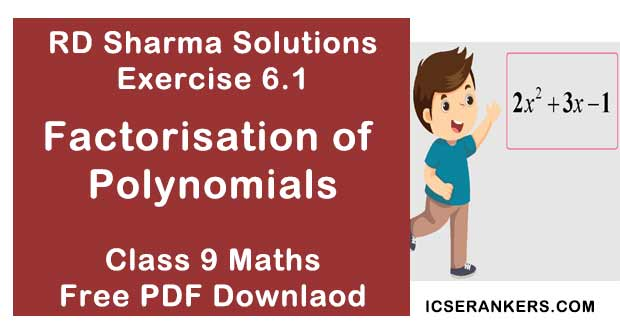# Chapter 6 Factorisation of Algebraic Expressions RD Sharma Solutions Exercise 6.1 Class 9 MathsChapter Name RD Sharma Chapter 6 Factorisation of Polynomials Exercise 6.1 Book Name RD Sharma Mathematics for Class 10 Other Exercises Exercise 6.2Exercise 6.3Exercise 6.4Exercise 6.5 Related Study NCERT Solutions for Class 10 Maths

### Exercise 6.1 Solutions

1. Which of the following expressions are polynomials in one variable and which are not ?
(i) 3x2 - 4x + 15
(ii) y2 + 2√3
(iii) 3√x + √2x
(iv) x - 4/x
(v) x12 + y3 + t50

Solution

(i) 3x2 - 4x + 15 is a polynomial of one variable x.
(ii) y2 + 2√3 is a polynomial of one variable y.
(iii) 3√x + √2x is not a polynomial as the exponents of 3√x is not a positive integer.
(iv) x - 4/x is not a polynomial as the exponent of -4/x is not a positive integer.
(v) x12 + y3 + t50 is a polynomial of three variables x, y, t.

2. Write the coefficient of x2 in each of the following:
(i) 17 - 2x + 7x2
(ii) 9 - 12x + x3
(iii) π/6 x2 - 3x + 4
(iv) √x - 7

Solution

Coefficient of x2 in
(i) 17 - 2x + 7x2 is 7
(ii) 9 - 12x + x3  is 0
(iii) π/6 x2 - 3x + 4 is π/6
(iv) √x - 7 is 0

3. Write the degree of each of the following polynomials :
(i) 7x3 + 4x2 - 3x + 12
(ii) 12 - x + 2x3
(iii) 5y - √2
(iv) 7 = 7 × x°
(v) 0

Solution

Degree of polynomial
(i) 7x3 + 4x2 - 3x + 12 is 3
(ii) 12 - x + 2x3 is  3
(iii) 5y - √2 is 1
(iv) 7 = 7 × x° is 0
(v) 0 is undefined.

4. Classify the following polynomials as linear, quadratic, cubic and biquadratic polynomials:
(i) x + x2 + 4
(ii) 3x - 2
(iii) 2x + x2
(iv) 3y
(v) t2 + 1
(vi) 7t4 + 4t3 + 3t - 2

Solution

Given polynomial
(i) x + x2 + 4 is quadratic as degree of polynomial is 2.
(ii) 3x - 2  linear as degree of polynomial is 1.
(iii) 2x + x2 is quadratic as degree of polynomial is 2.
(iv) 3y is linear as degree of polynomial is 2.
(v) t2 + 1 is quadratic as degree of polynomial is 2.
(vi) 7t4 + 4t3 + 3t - 2 is bi- quadratic as degree of polynomial is 4.

5. Classify the following polynomials as polynomials is one - variable, two variables etc:
(i) x2 - xy + 7y2
(ii) x2 - 2tx + 7t2 - x + t
(iii) t3 - 3t2 + 4t - 5
(iv) xy + yz + zx

Solution

(i) x2 - xy + 7y2  is a polynomial in two variables x, y.
(ii) x2 - 2tx + 7t2 - x + t is a polynomial in 2 variables x, t.
(iii) t3 - 3t2 + 4t - 5 is a polynomial in 1 variables t.
(iv) xy + yz + zx is a polynomial in 3 variables x, y, z.

6. Identify polynomials in the following:
(i) f(x) = 4x2 - x2 - 3x + 7
(ii) g(x) = 2x3 - 3x2 + √x - 1
(iii) p(x) = 2/3 x2 - 7/4 x + 9.
(iv) q(x) = 2x2 - 3x + 4/x + 2
(v) h(x) = x4 - x3/2 + x - 1
(vi) f(x) = 2 + 3/x + 4x

Solution

(i) f(x) = 4x2 - x2 - 3x + 7 is a polynomial
(ii) g(x) = 2x3 - 3x2 + √x - 1 is not a polynomial as exponent of x in √x is not a positive integer.
(iii) p(x) = 2/3 x2 - 7/4 x + 9.  is a polynomial as all the exponents are positive integers.
(iv) q(x) = 2x2 - 3x + 4/x + 2 is not a polynomial as exponent of x in 4/x is not a positive integer.
(v) h(x) = x4 - x3/2 + x - 1 is not a polynomial as exponent of x in  - x3/2 is not a positive integer.
(vi) f(x) = 2 + 3/x + 4x  is not a polynomial as exponent of x in 3/x is not a positive integer.

7. Identify constant, linear, quadratic and cubic polynomials from the following polynomials:
(i) f(x) = 0
(ii) g(x) = 2x3 - 7x + 4
(iii) h(x) = -3x + 1/2
(iv) p(x) = 2x3 - x + 4
(v) q(x) = 4x + 3
(vi) r(x) = 3x2 + 4x2 + 5x - 7

Solution

Given polynomial
(i) f(x) = 0  is a constant polynomial as 0 is a constant
(ii) g(x) = 2x3 - 7x + 4 is a cubic polynomial as degree of the polynomial is 3.
(iii) h(x) = -3x + 1/2 is a linear polynomial as degree of the polynomial is  1.
(iv) p(x) = 2x3 - x + 4  is a quadratic as the degree of the polynomial is 2.
(v) q(x) = 4x + 3  is a linear polynomial as the degree of the polynomial is 1.
(vi) r(x) = 3x2 + 4x2 + 5x - 7 is a cubic polynomial as the degree is 3.

8. Give one example each of a binomial of degree 35, and of a monomial of degree 100.

Solution

Example of a binomial with degree 35 is 7x35 - 5.
Example of a monomial with degree 100 is 2t100